Next: Special Cases: Supernova Explosion Up: Change of Binary Parameters: Previous: Mass change

Semi-major axis change

The binary separation a changes differently depending on the mass exchange mode. First, we introduce a measure of non-conservativeness of the mass exchange in the form of the ratio between the accreting and the mass-loosing star mass changesIf the mass transfer is conservative (, i.e.const) and one may neglect the redistribution of the intrinsic angular momenta of the components, the total orbital momentum conservation law impliesIn a more general case of quasi-conservative mass transfer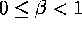, the orbital separation changes depend on the specific angular momentum removed from the system by the escaping matter (see detailed discussion in van den Heuvel (1994)). To be specific, we use the ``isotropic mass loss mode''Ā by letting matter remove the specific orbital angular momentum of the accreting component (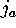)from which we straightforwardly find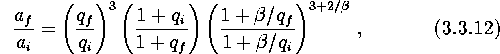here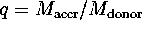.

If no matter is trapped by the secondary companion without additional sinks of angular momentum (the so-called absolutely non-conservative case), which relates to the spherically symmetric stellar windĀ from one component, we use another well-known formula(the orbital separation always increases in such systems).

When the orbital angular momentum is removed by GR or MSW with no RL overflow, the following approximate formulas are used:In a special case of a WD filling its RL (stage ``IIIwd'' above) under assumption of a stable conservative mass transfer and taking into account that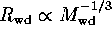, it can be shown that the orbital separation must increaseMike E. Prokhorov
Sat Feb 22 18:38:13 MSK 1997